Next: Theorems Up: k-Fibonacci-q numbers and new Previous: k-Fibonacci-q numbers and new   Contents

## Definitions

• The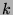-Fibonacci-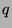sequence (the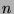th term of which is denoted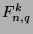) is defined recursively by the following statements:
• If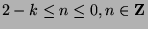, then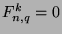.
•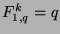.
•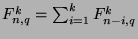,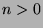.
Note that this idea encompasses both the-Fibonacci sequence and the Fibonacci-sequence.
•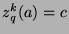means that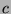has the following properties:
•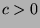.
• For all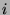such that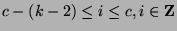, it follows that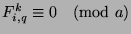.
•is the least positive integer satisfying these properties.
•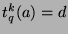means that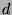has the following properties:
•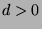• For allsuch that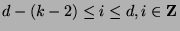, it follows that.
•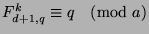.
•is the least positive integer satisfying these properties.
Note: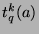is then the function that gives the period of the-Fibonacci-sequence in modulo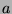.
•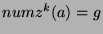means that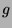has the following properties:
•is the number ofthat satisfy the property that allfrom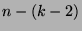to, inclusive, satisfy the property that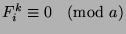, wheregoes from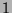to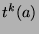, inclusive.
•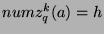means that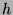has the following properties:
•is the number ofthat satisfy the property that allfromto, inclusive, satisfy the property that, wheregoes fromto, inclusive.Next: Theorems Up: k-Fibonacci-q numbers and new Previous: k-Fibonacci-q numbers and new   Contents
Gregory Stoll 2000-04-08